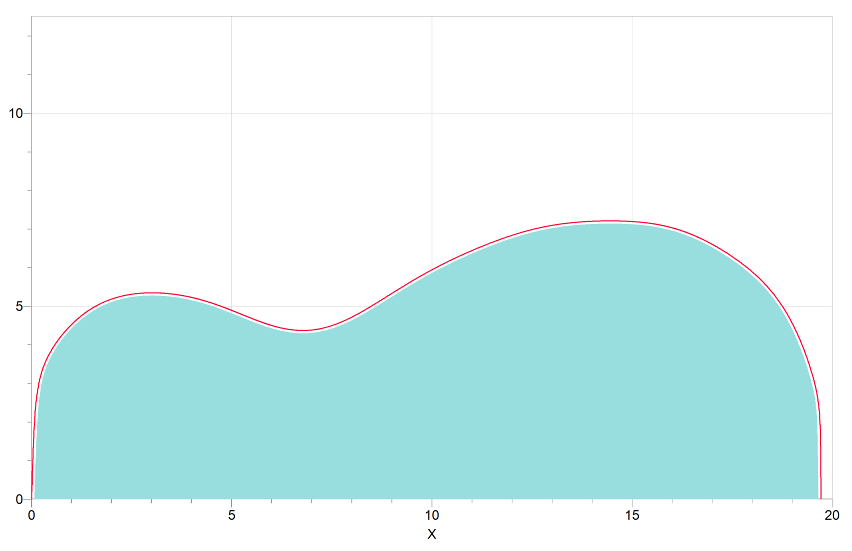Red          Outside curve Green      Inside Area
 Graphed in Logger Pro
 Beard Resonator Inside Area = 108.3 square inches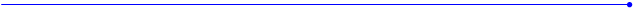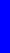The Nealon Equationy  =  (A*sin(B*x+C) + D*sin(E*x+F) + G*sin(H*x+I) + J*sin(K*x+L)) *  (M + N*x + O*x^2 + P*x^3 + Q*x^4) * atan(R*x) * atan(S*(BL-x))Beard Resonator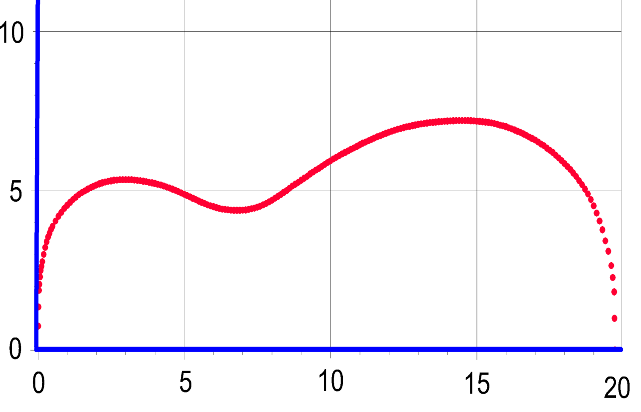Graphed in Logger Pro
 Data from blueprint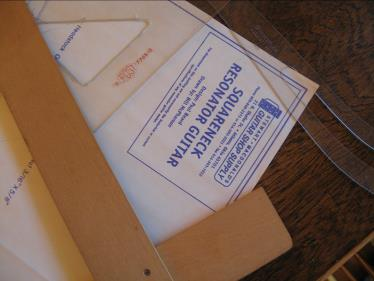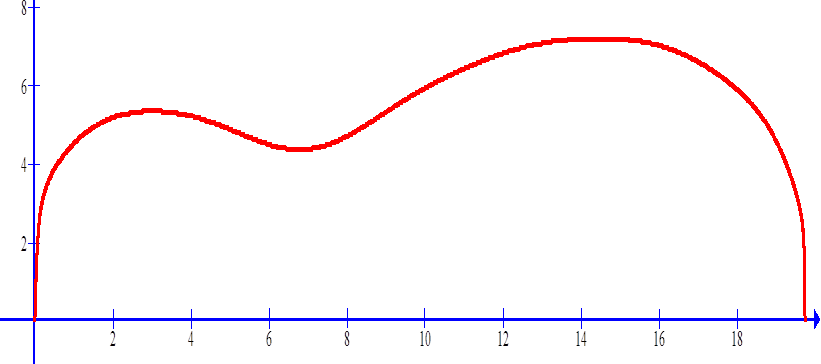Calculated curve
 Graphed in “Graph”
 y = (A*sin(B*x+C)+D*sin(E*x+F)+G*sin(H*x+I)+J*sin(K*x+L))*(M+N*x+O*x^2+P*x^3+Q*x^4)*atan(R*x)*atan(S*(19.725-x))This is the Beard square neck resonator in Graph where y(x) and – y(x) are plotted.  The axes are removed.webpages and Eos image copyright  2014  M  Nealon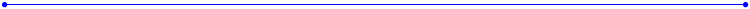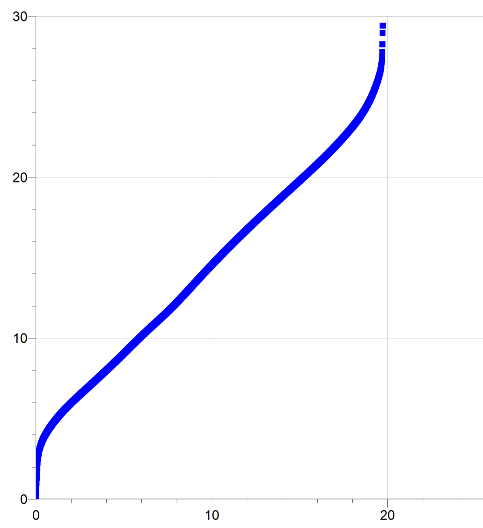Total length of side  =  29.4 inches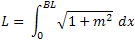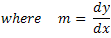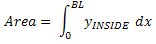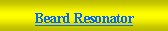Beard Resonator A 0.09921364 B -1.205259 C 0.0986698 D 0.4959425 E 0.8388244 F 5.147737 G 6.057618 H 0.108849 I 3.936981 J 2.265881 K 0.4181337 L -4.280678 M -0.5284347 N -0.0591946 O 0.04773545 P -0.005983448 Q 0.000189199 R 16.59738 S 71.12232 BL 19.725 A B C D E F G H I J K L M N O P Q R S BL 0.09921364 -1.205259 0.0986698 0.4959425 0.8388244 5.147737 6.057618 0.108849 3.936981 2.265881 0.4181337 -4.280678 -0.5284347 -0.0591946 0.04773545 -0.005983448 0.000189199 16.59738 71.12232 19.725 A = 0.09921364 B = -1.205259 C = 0.0986698 D = 0.4959425 E = 0.8388244 F = 5.147737 G = 6.057618 H = 0.108849 I = 3.936981 J = 2.265881 K = 0.4181337 L = -4.280678 M = -0.5284347 N = -0.0591946 O = 0.04773545 P = -0.005983448 Q = 0.000189199 R = 16.59738 S = 71.12232 BL = 19.725